/

### The Clayton copula

The Clayton copula is a copula that allows any specific non-zero level of (lower) tail dependency between individual variables. It is an Archimedean copula, and exchangeable.

 Copula name Clayton copula Common notation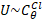Parameters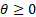(can be extended to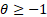) Domain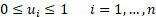Copula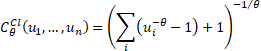Or if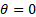we use the limit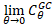Kendall’s rank correlation coefficient (for bivariate case)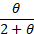Coefficient of upper tail dependence,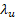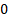Coefficient of lower tail dependence,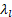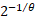Archimedean generator function,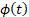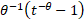Or ifwe use the limit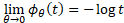. If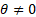then a simpler version, which does not alter the copula itself, is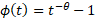. Other comments Ifwe obtain the independence copula. The Clayton copula (like the Frank copula) is a comprehensive copula in that it interpolates between a lower limit of the countermonotonicity copula (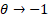) and an upper limit of the comonotonicity copula (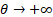)..

Nematrian web functions

Functions relating to the above distribution may be accessed via the Nematrian web function library by using a DistributionName of “Clayton Copula”. For details of other probability distributions see here.